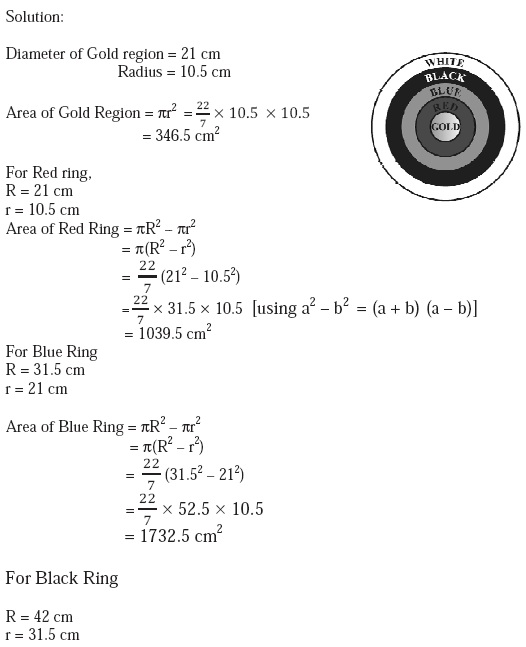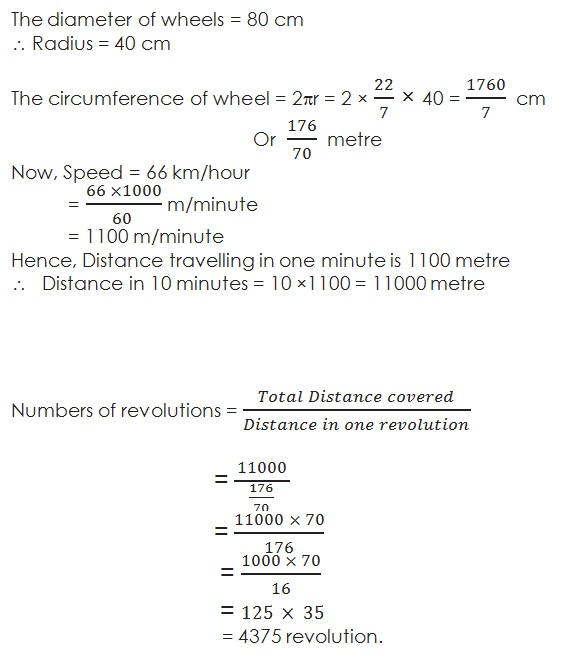Study Materials

NCERT Solutions for Class 10th Mathematics

Page 1 of 4

Chapter 12. Areas Related to Circles

Exercise: 12.1

Q.1   The radii of two circles are 19 cm and 9 cm respectively. Find the radius of the circle which has circumference equal to the sum of the circumferences of the two circles.

Solution:

Let the radius of acquired circle = R

And radius of Large circle r1 = 19 cm

Radius of small circle r2 = 9 cm

Then,

2πR = 2πr1 + 2πr2

2πR = 2π (r1 + r2)

R = (r1 + r2)

= 19 + 9

= 28 cm

Q2.   The radii of two circles are 8 cm and 6 cm respectively. Find the radius of the circle having area equal to the sum of the areas of the two circles.

Solution:

Let the radius of acquired circle = R

And radius of Large circle r1 = 8 cm

Radius of small circle r2 = 6 cm

Then,

πR2 = πr12 + πr22

πR2 = π (r12 + r22)

R2 = (r12 + r22)

= 82 + 62

= 64 + 36 cm

= 100 cm

R = √100 = 10 cm

Q3.   Depicts an archery target marked with its five scoring areas from the centre outwards as Gold, Red, Blue, Black and White. The diameter of the region representing Gold score is 21 cm and each of the other bands is 10.5 cm wide. Find the area of each of the five scoring regions.Q4.  The wheels of a car are of diameter 80 cm each. How many complete revolutions does each wheel make in 10 minutes when the car is travelling at a speed of 66 km per hour?

Solution:Q5.   If the perimeter and the area of a circle are numerically equal, then find the radius of the circle.

Solution:

2πr = πr2

2r  = r2

2r  = r × r

r = 2 unit

Page 1 of 4

Chapter Contents: